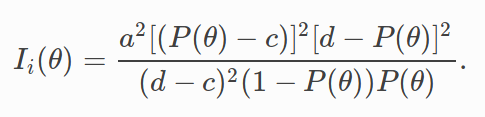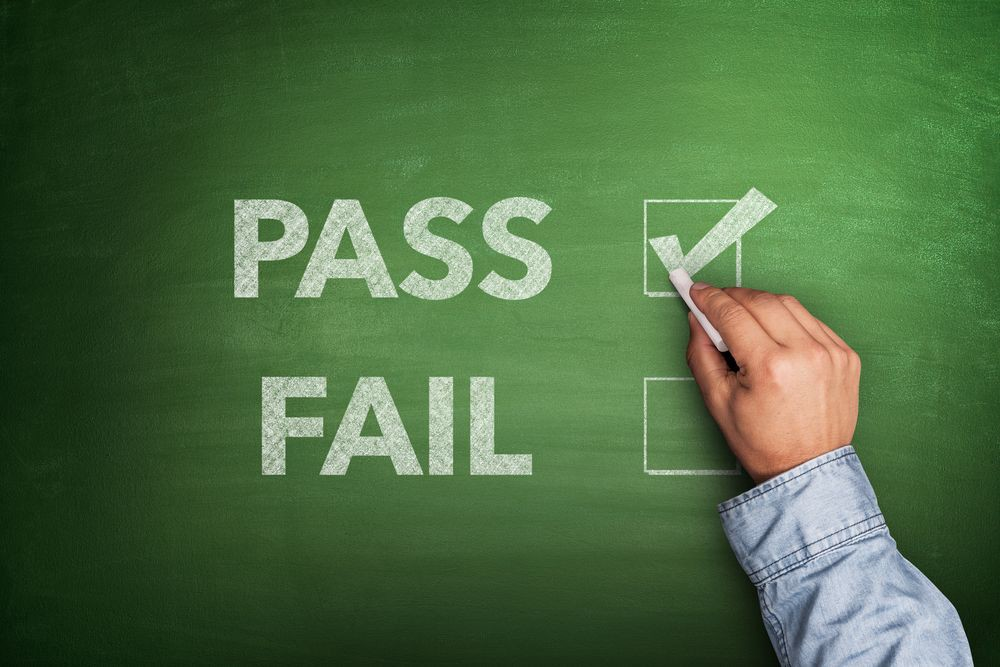In this post, I’ll share the step by step process we used at AirCTO to create computerized adaptive tests.

At AirCTO we use adaptive tests to measure the ability of the candidate in different domains (Python, Front end development, Docker etc,.).

### Back to basics: What is computerized adaptive test?#

Computerized adaptive testing (CAT)) is a form of computer-based test that adapts to the examinee’s ability level. For this reason, it has also been called tailored testing. In other words, it is a form of computer-administered test in which the next item or set of items selected to be administered depends on the correctness of the test taker’s responses to the most recent items administered.## Why we use the adaptive test?#

Adaptive tests are designed to challenge candidates. High-achieving candidates who take a test that is designed around an average are not challenged by questions below their individual achievement abilities.

Likewise, if lower-achieving candidates served by questions that are far above their current abilities they are left guessing the answers instead of applying what they already know.Adaptive testing addresses these issues by adjusting the questions to the individual proficiency of the candidate. High-achieving candidates are challenged by more difficult questions, while candidates who are slightly below the average are not overwhelmed but rather encouraged to continue moving forward by answering questions at or slightly above their current achievement level.

• This motivates candidates to reach slightly beyond their comfort zone no matter where that zone might be-and prevents them from getting discouraged.

• The adaptive test is based on a statistical model defined by the Item Response Theory.

• We measure the ability of the candidate and the question difficulty on the same scale.

True Score/ True Ability: True score is the score an examinee would receive on a perfectly reliable test. Since all tests contain error, true scores are a theoretical concept; in an actual testing program, we will never know an individual’s true score.

We can however, compute an estimate of an examinee’s true score and we can estimate the amount of error in that estimate. True ability is denoted as θ; the true score for examinee j is denoted θ-j.

## Item Response Theory (IRT):#

Item Response Theory (IRT) is a statistical framework in which examinees can be described by a set of one or more ability scores that are predictive, through mathematical models, linking actual performance on test items, item statistics, and examinee abilities.

IRT states that the probability of an examinee correctly answering the question is a function of the candidates true ability (θ) and the difficulty of the question (bi).Source

Under the 3 parameter IRT model, the probability of a correct response to a given item is a function of an examinee’s true ability and three item parameters:

# Item parameters:#

The parameters are calculated based on prior administrations of the items to a sample population. This is called pilot-test. It is possible to calibrate item parameters from the responses on a test.

Initial item parameters are selected by modeling IRT parameters with responses from a sample population.

## 1. `ai` : Item Discrimination Parameter#

This parameter shows how well an item (question) discriminates individuals who answer the item correctly and those who don’t.

An item with a high value tends to be answered correctly by all individuals whose θ is above the items' difficulty level and wrongly by all the others.

## 2. `bi` : Item Difficulty Parameter#

`b` represents an item’s `difficulty parameter`. This parameter is measured on the same scale as θ. It shows at which point of the proficiency scale the item is more is informative, that is, where it discriminates the individuals who agree and those who disagree with the item.

Since b and θ are measured in the same scale, b follows the same distributions as θ.

For a CAT, it is good for an item bank to have as many items as possible in all difficulty levels, so that the CAT may select the best item for each individual in all ability levels.

## 3. `ci` : Guessing parameter#

`c` represents an item’s `pseudo-guessing parameter`. This parameter denotes what is the probability of individuals with low proficiency values to answer the item correctly. Since c is a probability, 0< c ≤1, the lower the value of this parameter, the better the item is considered.

Let’s assume that you are given a question with four options and you do know the correct answer. If you select an answer randomly there is a 0.25 chance of success. This is the guessing parameter.

Some times when you know the answer partially, one question might feel more probable than others. In this case the guessing parameter will be the highest probable one.

## 4. `di` : Upper asymptote#

An asymptote of a curve is a line such that the distance between the curve and the line approaches zero as one or both of the x or y coordinates tends to infinity.`d` represents an item’s `upper asymptote`. This parameter denotes what is the probability of individuals with high proficiency values to still answer the item incorrectly.

Since d is a probability, 0< d ≤1, the higher the value of this parameter, the better the item is considered.

In our implementation, we have used three-parameter model (3PL) with parameters `a, b, c and d` is a constant numpy.ones((n)).Each item has a different set of these three parameters. These parameters are usually calculated based on prior administrations of the item.

You can simulate these parameters using the following probability distributions:

``````discrimination: N(1.2,0.25) difficulty: N(0,1)
pseudo_guessing: N(0.25,0.02) upper asymptote: N(0.93,0.02)
``````Once we have enough samples these item parameters can be re-calibrated for better performance. θ is the examinee’s true ability, θ^ is the estimated ability.

The 3PL IRT model states that probability of a correct response to an item i for an examinee is a function of the three item parameters and examinee j’s true ability θj.

Under IRT, the probability of an examinee with a given θ^ value (estimated ability), to answer item i correctly, given the item parameters, is given by:With IRT, maximum information can be quantified as the standardized slope of ( θ) at θ^ . In other wordsThe CAT (Computerized Adaptive Test) algorithm: is usually an iterative process with the following steps:

• Ability Estimation: All the items that have not yet been administered are evaluated to determine which will be the best one to administer as next-item, given the currently estimated ability level.

• Item Selection: The “best” next item is administered and the examinee responds.

• A new ability estimate is computed based on the responses to all of the administered items.`(Log likelyhood, DifferentialEvolutionEstimator, HillClimbingEstimator)`

• Steps 1 through 3 are repeated until a stopping criterion is met.Ability Initialisation: (only first time) We can initialize Randomly or on Fixed Point (-4, 4).

Ability estimation: (except first time): A new ability estimate is computed based on the responses to all of the administered items. There are two main types of ways of estimating θ^: and these are the Bayesian methods and maximum-likelihood ones.

Maximum-likelihood methods choose the θ^ value that maximizes the log likelihood of an examinee having a certain response vector, given the corresponding item parameters.

Bayesian methods used a priori information (usually assuming proficiency and parameter distributions) to make new estimations. The knowledge of new estimations is then used to make new assumptions about the parameter distributions, refining future estimations. 

## Item Selection:#

We used an implementation of the random sequence selector in which, at every step of the test, an item is randomly chosen from the n most informative items in the item bank, n being a predefined value.

## Stopping rule:#

The stopping criterion could be time, the number of items administered, change in ability estimate, content coverage, a precision indicator such as the standard error.

We use a combination of : time, ability estimate and precision.

In other words:

With IRT, maximum information can be quantified as the standardised slope of Pi( theta ) at theta_hat.Cut Score Determination: While there are many methods for setting the cut score, there are a few that easily incorporate the information provided by IRT methods.

That is, many methods such as The θ values obtained via IRT provide a unique opportunity to get a more reliable estimate of respondent scores, and you are wise to look for a cut score method that can incorporate that info and typically use observed scores for determining the cut score, and inherently ignore the measurement error involved in that. in IRT: Pass/Fail

The adaptive test will give the ability of the user in the interval -4 to +4 (low, high). We can either use this scale or convert it to a different scale: Transform your data using a desired mean and standard deviation.